Anzeige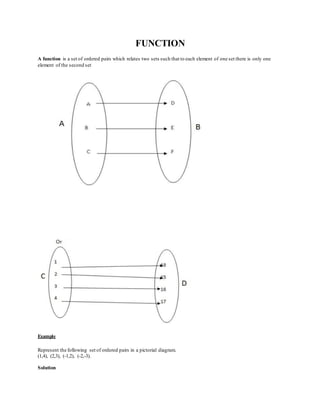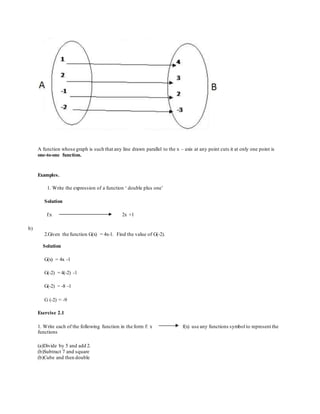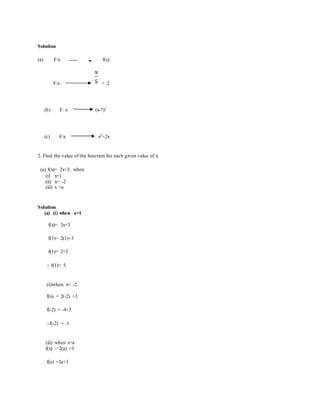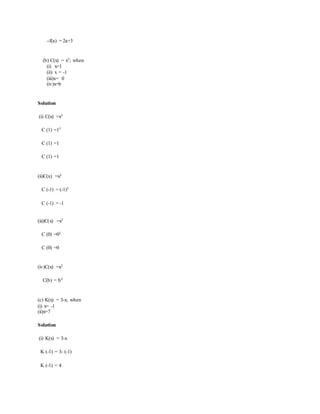Anzeige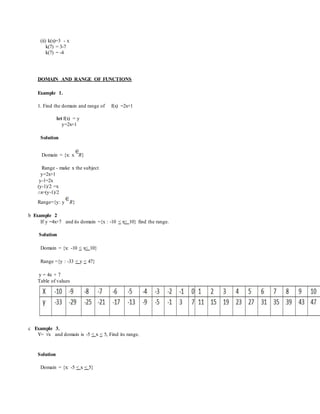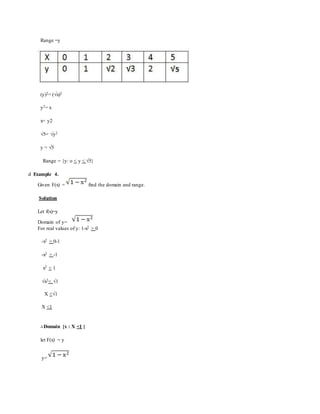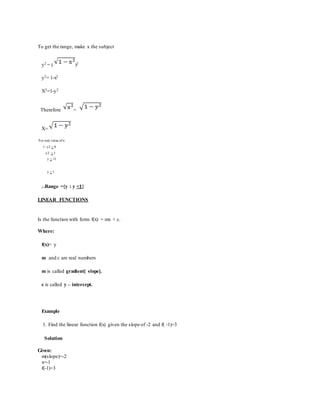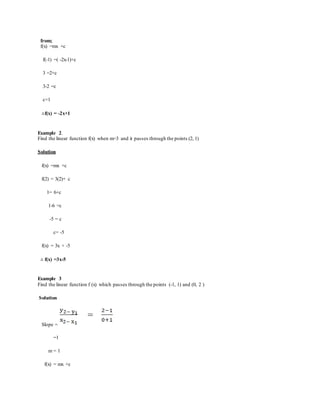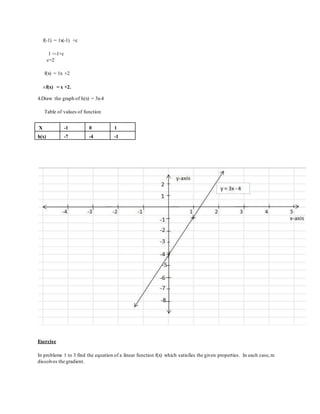Anzeige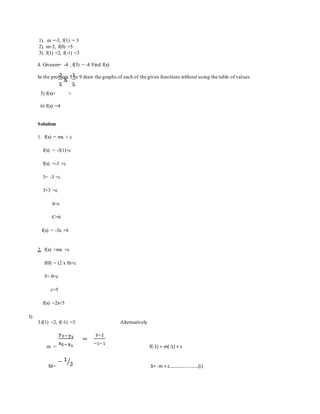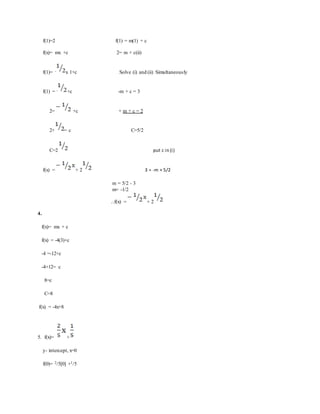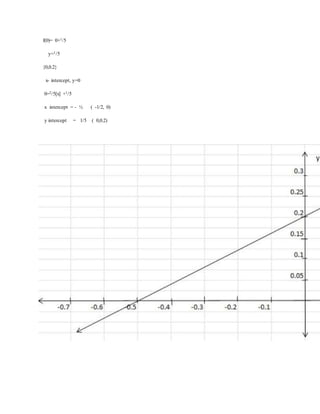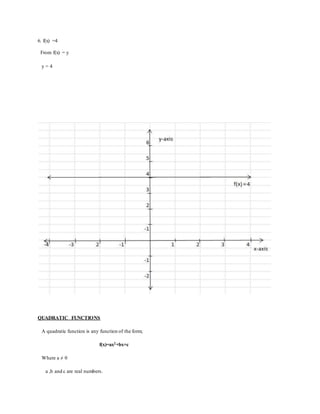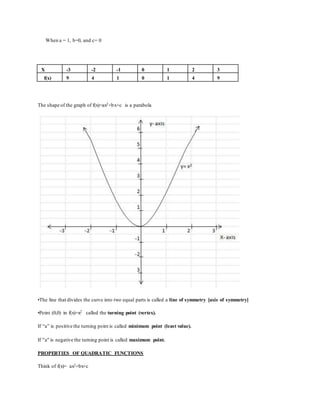Anzeige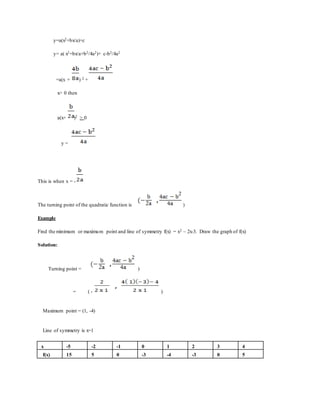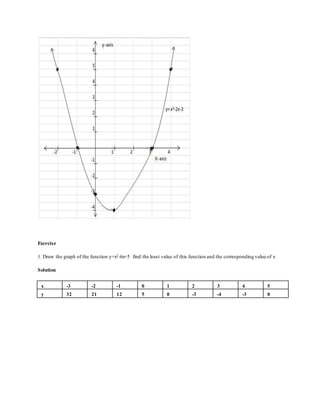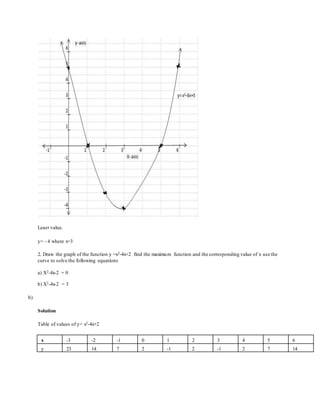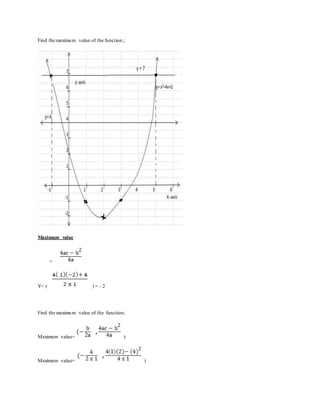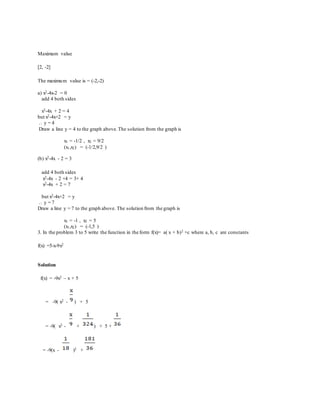Anzeige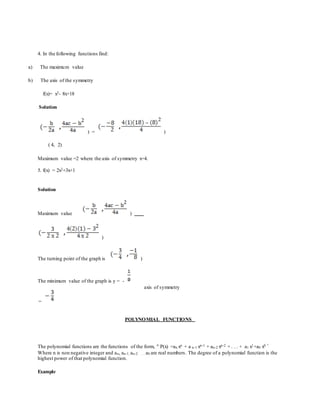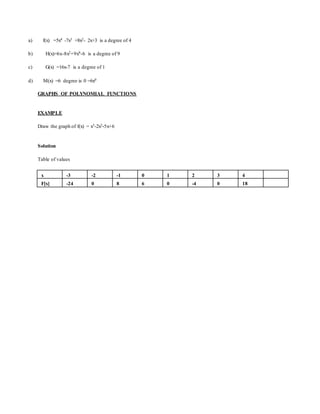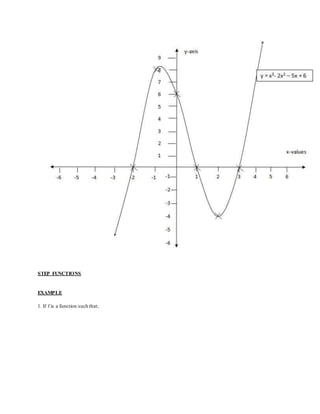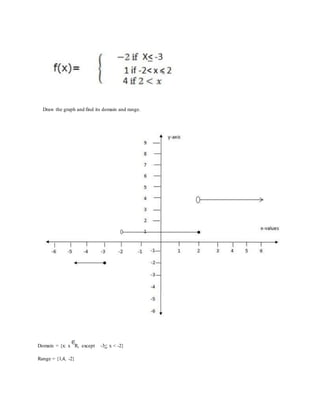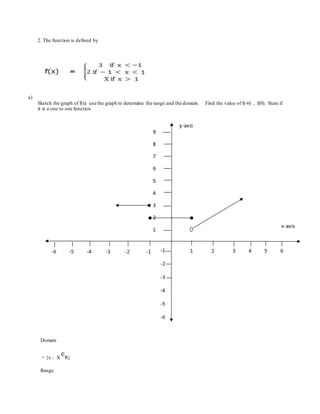Anzeige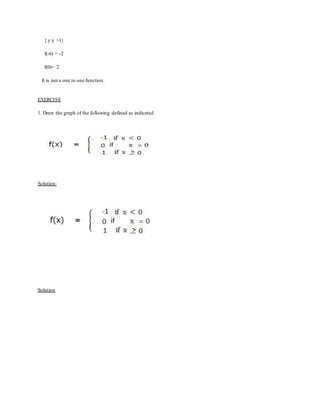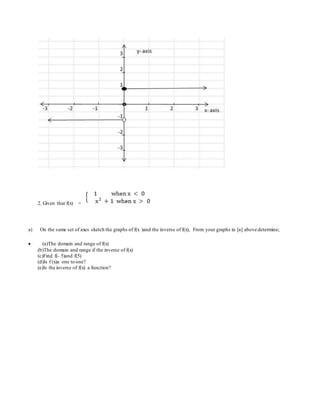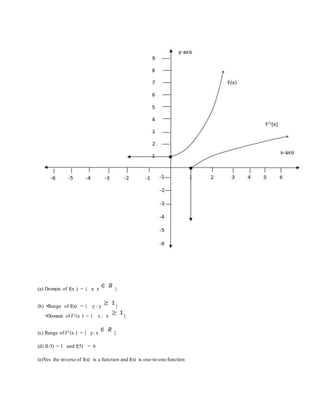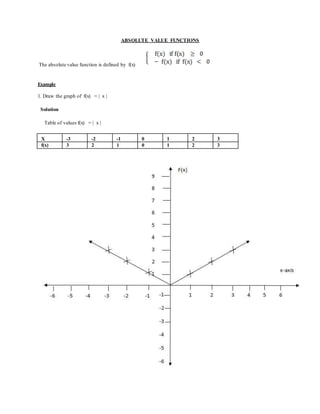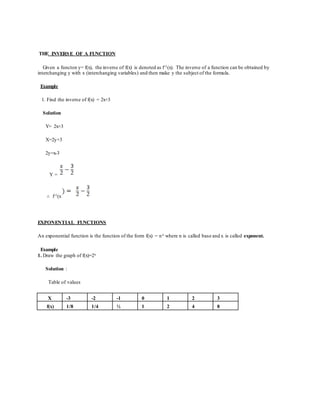Anzeige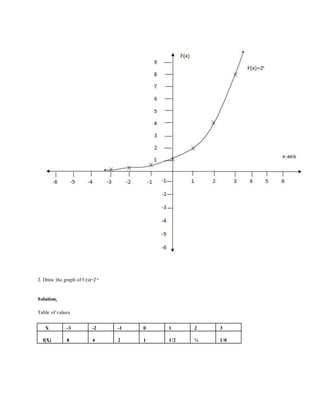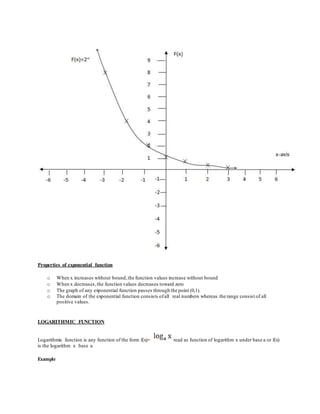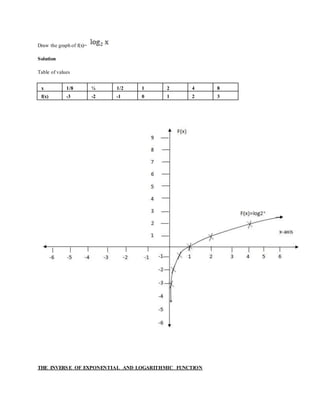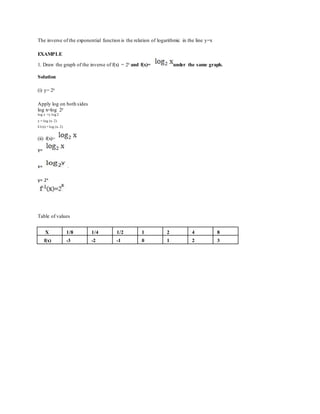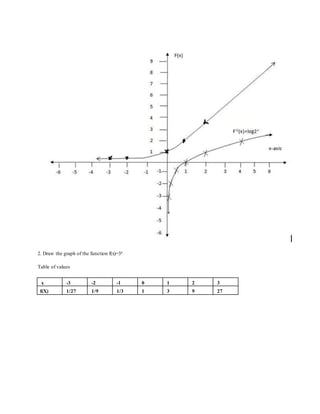Anzeige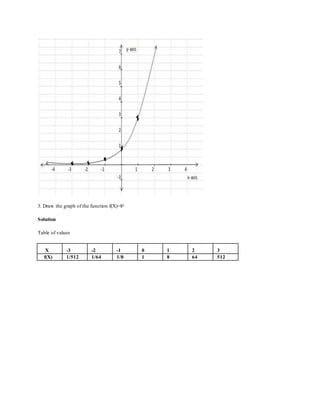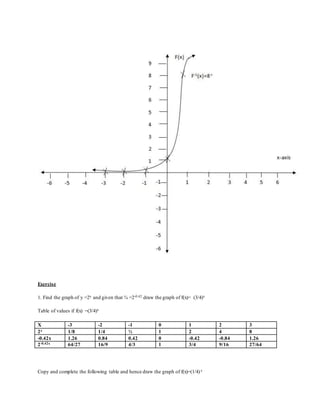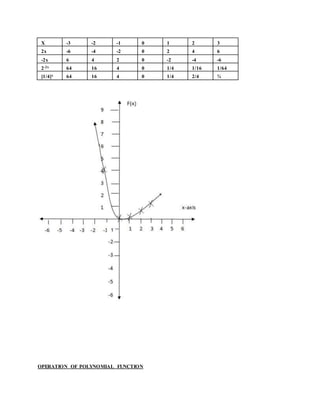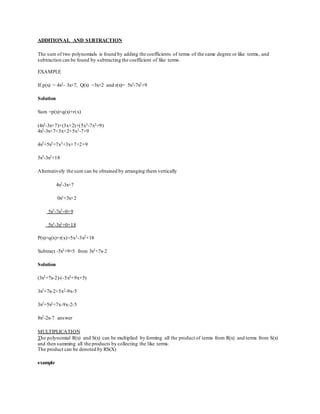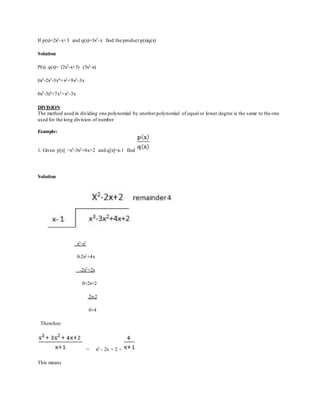Anzeige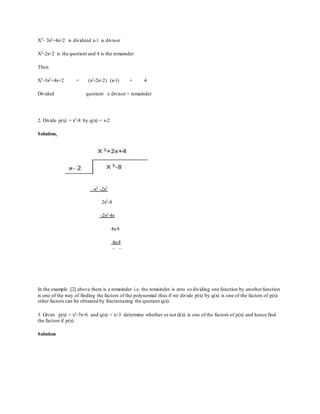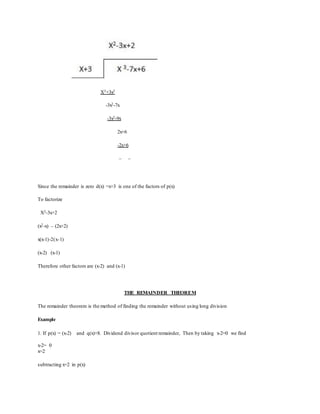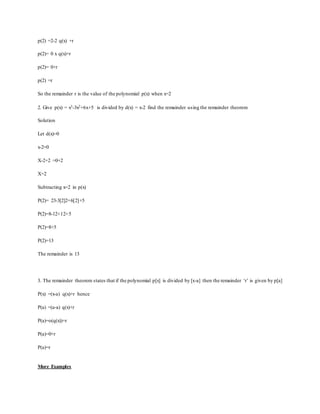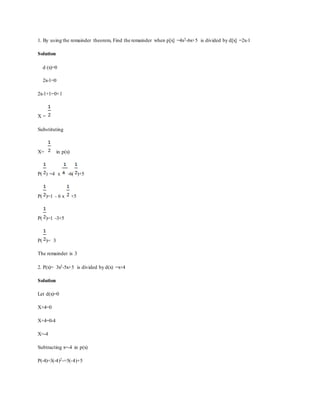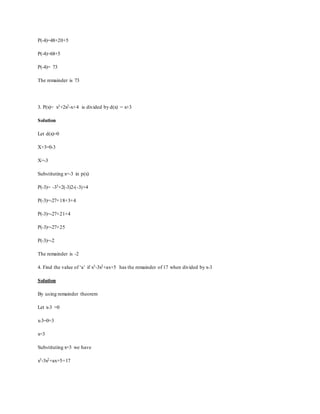Anzeige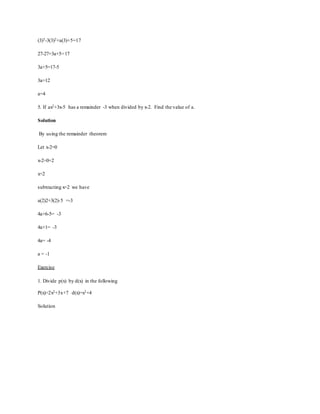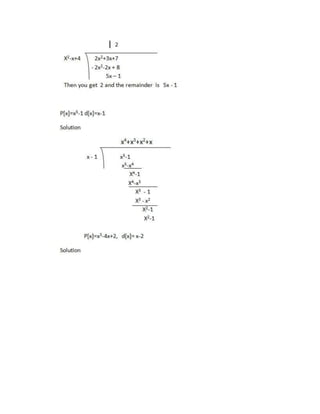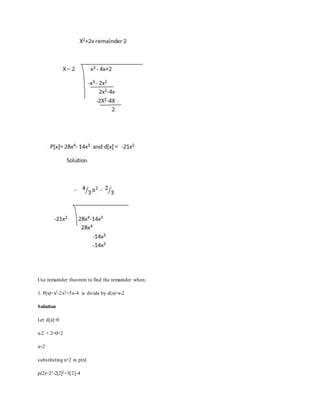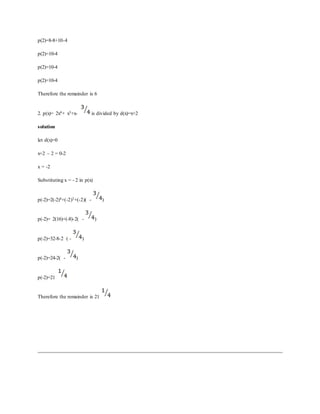Nächste SlideShare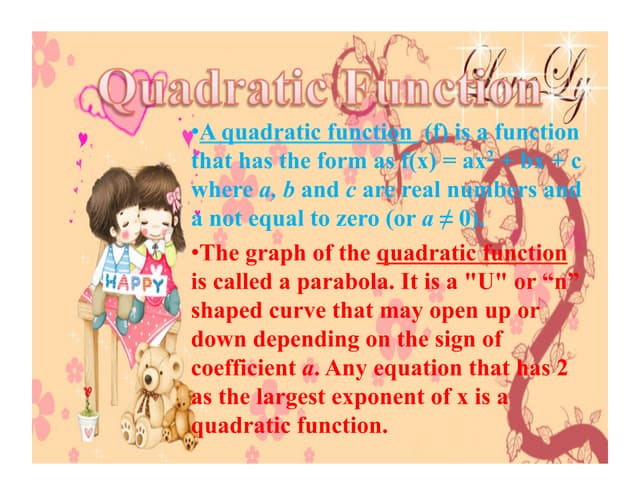Quadratic Function Presentation
1 von 48
Anzeige

### Function

1. FUNCTION A function is a set of ordered pairs which relates two sets such that to each element of one set there is only one element of the second set Example Represent the following set of ordered pairs in a pictorial diagram. (1,4), (2,3), (-1,2), (-2,-3). Solution
2. A function whose graph is such that any line drawn parallel to the x – axis at any point cuts it at only one point is one-to-one function. Examples. 1. Write the expression of a function ‘ double plus one’ Solution f:x 2x +1 b) 2.Given the function G(x) = 4x-1. Find the value of G(-2). Solution G(x) = 4x -1 G(-2) = 4(-2) -1 G(-2) = -8 -1 G (-2) = -9 Exercise 2.1 1. Write each of the following function in the form f: x f(x) use any functions symbol to represent the functions (a)Divide by 5 and add 2. (b)Subtract 7 and square (b)Cube and then double
3. Solution (a) F:x f(x) F:x + 2 (b) F: x (x-7)2 (c) F:x x3+2x 2. Find the value of the function for each given value of x. (a) f(x)= 2x+3; when (i) x=1 (ii) x= -2 (iii) x =a Solution (a) (i) when x=1 f(x)= 2x+3 f(1)= 2(1)+3 f(1)= 2+3 ∴ f(1)= 5. (ii)when x= -2. f(x) = 2(-2) +3 f(-2) = -4+3 ∴f(-2) = -1 (iii) when x=a f(x) = 2(a) +3 f(a) =2a+3
4. ∴f(a) = 2a+3 (b) C(x) = x3; when (i) x=1 (ii) x = -1 (iii)x= 0 (iv)x=b Solution (i) C(x) =x3 C (1) =13 C (1) =1 C (1) =1 (ii)C(x) =x3 C (-1) = (-1)3 C (-1) = -1 (iii)C(x) =x3 C (0) =03 C (0) =0 (iv)C(x) =x3 C(b) = b3 (c) K(x) = 3-x; when (i) x= -1 (ii)x=7 Solution (i) K(x) = 3-x K (-1) = 3- (-1) K (-1) = 4
5. (ii) k(x)=3 - x k(7) = 3-7 k(7) = -4 DOMAIN AND RANGE OF FUNCTIONS Example 1. 1. Find the domain and range of f(x) =2x+1 let f(x) = y y=2x+1 Solution Domain = {x: x R} Range - make x the subject y=2x+1 y-1=2x (y-1)/2 =x ∴x=(y-1)/2 Range={y: y R} b Example 2 If y =4x+7 and its domain ={x : -10 < x< 10} find the range. Solution Domain = {x: -10 < x< 10} Range ={y : -33 < y < 47} y = 4x + 7 Table of values c Example 3. Y= √x and domain is -5 < x < 5, Find its range. Solution Domain = {x: -5 < x < 5}
6. Range =y Table of value of x = √(y) (y)2= (√x)2 y2= x x= y2 √5= √y2 y = √5 Range = {y: o < y < √5} d Example 4. Given F(x) = find the domain and range. Solution Let f(x)=y Domain of y= For real values of y: 1-x2 > 0 -x2 > 0-1 -x2 > -1 x2 < 1 √x2< √1 X <√1 X <1 ∴Domain {x : X <1 } let F(x) = y y=
7. To get the range, make x the subject y2 = ( )2 y2= 1-x2 X2=1-y2 Therefore = X= For real value ofx: 1- y2 > 0 y2 < 1 y < √1 y < 1 ...Range ={y : y <1} LINEAR FUNCTIONS Is the function with form f(x) = mx + c. Where: f(x)= y m and c are real numbers m is called gradient[ slope]. c is called y – intercept. Example 1. Find the linear function f(x) given the slope of -2 and f( -1)=3 Solution Given: m(slope)=-2 x=-1 f(-1)=3
8. from; f(x) =mx +c f(-1) =( -2x-1)+c 3 =2+c 3-2 =c c=1 ∴f(x) = -2x+1 Example 2. Find the linear function f(x) when m=3 and it passes through the points (2, 1) Solution f(x) =mx +c f(2) = 3(2)+ c 1= 6+c 1-6 =c -5 = c c= -5 f(x) = 3x + -5 ∴ f(x) =3x-5 Example 3 Find the linear function f (x) which passes through the points (-1, 1) and (0, 2 ) Solution Slope = =1 m = 1 f(x) = mx +c
9. f(-1) = 1x(-1) +c 1 =-1+c c=2 f(x) = 1x +2 ∴f(x) = x +2. 4.Draw the graph of h(x) = 3x-4 Table of values of function Exercise In problems 1 to 3 find the equation of a linear function f(x) which satisfies the given properties. In each case,m dissolves the gradient. X -1 0 1 h(x) -7 -4 -1
10. 1). m =-3, f(1) = 3 2). m=2, f(0) =5 3). f(1) =2, f(-1) =3 4. Givenm= -4 , f(3) = -4 Find f(x) In the problem 5 to 9 draw the graphs of each of the given functions without using the table of values 5) f(x)= + 6) f(x) =4 Solution 1. f(x) = mx + c f(x) = -3(1)+c f(x) =-3 +c 3= -3 +c 3+3 =c 6=c C=6 f(x) = -3x +6 2. f(x) =mx +c f(0) = (2 x 0)+c 5= 0+c c=5 f(x) =2x+5 3) 3.f(1) =2, f(-1) =3 Alternatively m = f(-1) = m(-1) + c M= 3= -m + c.......................(i)
11. f(1)=2 f(1) = m(1) + c f(x)= mx +c 2= m + c(ii) f(1)= - x 1+c Solve (i) and (ii) Simultaneously f(1) = - +c -m + c = 3 2= +c + m + c = 2 2+ = c C=5/2 C=2 put c in (i) f(x) = + 2 3 = -m + 5/2 m = 5/2 - 3 m= -1/2 ...f(x) = + 2 4. f(x)= mx + c f(x) = -4(3)+c -4 =-12+c -4+12= c 8=c C=8 f(x) = -4x+8 5. f(x)= + y- intercept, x=0 f(0)= 2/5 +1/5
12. f(0)= 0+1/5 y=1/5 {0,0.2} x- intercept, y=0 0=2/5[x] +1/5 x intercept = - ½ ( -1/2, 0) y intercept = 1/5 ( 0,0.2)
13. 6. f(x) =4 From f(x) = y y = 4 QUADRATIC FUNCTIONS A quadratic function is any function of the form; f(x)=ax2+bx+c Where a ≠ 0 a ,b and c are real numbers.
14. When a = 1, b=0, and c= 0 X -3 -2 -1 0 1 2 3 f(x) 9 4 1 0 1 4 9 The shape of the graph of f(x)=ax2+bx+c is a parabola •The line that divides the curve into two equal parts is called a line of symmetry [axis of symmetry] •Point (0,0) in f(x)=x2 called the turning point (vertex). If “a” is positive the turning point is called minimum point (least value). If "a" is negative the turning point is called maximum point. PROPERTIES OF QUADRATIC FUNCTIONS Think of f(x)= ax2+bx+c
15. y=a(x2+bx/a)+c y= a( x2+bx/a+b2/4a2)+ c-b2/4a2 =a(x + ) 2 + x> 0 then a(x+ )2 > 0 y = This is when x = - The turning point of the quadratic function is ) Example Find the minimum or maximum point and line of symmetry f(x) = x2 – 2x-3. Draw the graph of f(x) Solution: Turning point = ) = ( - ) Maximum point = (1, -4) Line of symmetry is x=1 x -5 -2 -1 0 1 2 3 4 f(x) 15 5 0 -3 -4 -3 0 5
16. Exercise 1. Draw the graph of the function y=x2-6x+5 find the least value of this function and the corresponding value of x Solution x -3 -2 -1 0 1 2 3 4 5 y 32 21 12 5 0 -3 -4 -3 0
17. Least value. y= - 4 where x=3 2. Draw the graph of the function y =x2-4x+2 find the maximum function and the corresponding value of x use the curve to solve the following equations a) X2-4x-2 = 0 b) X2-4x-2 = 3 b) Solution Table of values of y= x2-4x+2 x -3 -2 -1 0 1 2 3 4 5 6 y 23 14 7 2 -1 2 -1 2 7 14
18. Find the maximum value of the function.; Maximum value = Y= ( ) = - 2 Find the maximum value of the function; Maximum value= ) Maximum value= )
19. Maximum value [2, -2] The maximum value is = (-2,-2) a) x2-4x-2 = 0 add 4 both sides x2-4x + 2 = 4 but x2-4x+2 = y ... y = 4 Draw a line y = 4 to the graph above.The solution from the graph is x1 = -1/2 , x2 = 9/2 (x1,x2) = (-1/2,9/2 ) (b) x2-4x - 2 = 3 add 4 both sides x2-4x - 2 +4 = 3+ 4 x2-4x + 2 = 7 but x2-4x+2 = y ... y = 7 Draw a line y = 7 to the graph above. The solution from the graph is x1 = -1 , x2 = 5 (x1,x2) = (-1,5 ) 3. In the problem 3 to 5 write the function in the form f(x)= a( x + b)2 +c where a, b, c are constants f(x) =5-x-9x2 Solution f(x) = -9x2 – x + 5 = -9( x2 - ) + 5 = -9( x2 - + ) + 5 + = -9(x - )2 +
20. 4. In the following functions find: a) The maximum value b) The axis of the symmetry f(x)= x2- 8x+18 Solution ) = ) ( 4, 2) Maximum value =2 where the axis of symmetry x=4. 5. f(x) = 2x2+3x+1 Solution Maximum value ) ) The turning point of the graph is ) The minimum value of the graph is y = - axis of symmetry = POLYNOMIAL FUNCTIONS The polynomial functions are the functions of the form, " P(x) =an xn + a n-1 xn-1 + an-2 xn-2 + . . . + a1 x1+a0 x0 ". Where n is non negative integer and an, an-1, an-2 . . . a0 are real numbers. The degree of a polynomial function is the highest power of that polynomial function. Example
21. a) f(x) =5x4 -7x3 +8x2- 2x+3 is a degree of 4 b) H(x)=6x-8x2+9x9-6 is a degree of 9 c) G(x) =16x-7 is a degree of 1 d) M(x) =6 degree is 0 =6x0 GRAPHS OF POLYNOMIAL FUNCTIONS EXAMPLE Draw the graph of f(x) = x3-2x2-5x+6 Solution Table of values x -3 -2 -1 0 1 2 3 4 F[x] -24 0 8 6 0 -4 0 18
22. STEP FUNCTIONS EXAMPLE 1. If f is a function such that;
23. Draw the graph and find its domain and range. Domain = {x: x R, except -3< x < -2} Range = {1,4, -2}
24. 2. The function is defined by a) Sketch the graph of f(x) use the graph to determine the range and the domain. Find the value of f(-6) , f(0). State if it is a one to one function Domain = {x : X R} Range
25. { y:y >1} f(-6) = -2 f(0)= 2 It is not a one to one function. EXERCISE 1. Draw the graph of the following defined as indicated Solution: Solution
26. 2. Given that f(x) = a) On the same set of axes sketch the graphs of f(x )and the inverse of f(x), From your graphs in [a] above determine;  (a)The domain and range of f(x) (b)The domain and range if the inverse of f(x) (c)Find f(- 5)and f(5) (d)Is f (x)a one to one? (e)Is the inverse of f(x) a function?
27. (a) Domain of f(x ) = { x: x } (b) •Range of f(x) = { y : y } •Domain of f-1(x ) = { x : x } (c) Range of f-1(x ) = { y: x } (d) f(-5) = 1 and f(5) = 6 (e)Yes the inverse of f(x) is a function and f(x) is one-to-one function
28. ABSOLUTE VALUE FUNCTIONS The absolute value function is defined by f(x) Example 1. Draw the graph of f(x) = | x | Solution Table of values f(x) = | x | X -3 -2 -1 0 1 2 3 f(x) 3 2 1 0 1 2 3
29. THE INVERSE OF A FUNCTION Given a functon y= f(x), the inverse of f(x) is denoted as f-1(x). The inverse of a function can be obtained by interchanging y with x (interchanging variables) and then make y the subject of the formula. Example 1. Find the inverse of f(x) = 2x+3 Solution Y= 2x+3 X=2y+3 2y=x-3 Y = ∴ f-1(x EXPONENTIAL FUNCTIONS An exponential function is the function of the form f(x) = nx where n is called base and x is called exponent. Example 1. Draw the graph of f(x)=2x Solution : Table of values X -3 -2 -1 0 1 2 3 f(x) 1/8 1/4 ½ 1 2 4 8
30. 2. Draw the graph of f (x)=2-x Solution: Table of values X -3 -2 -1 0 1 2 3 f(X) 8 4 2 1 1/2 ¼ 1/8
31. Properties of exponential function o When x increases without bound,the function values increase without bound o When x decreases,the function values decreases toward zero o The graph of any exponential function passes through the point (0,1). o The domain of the exponential function consists ofall real numbers whereas the range consist of all positive values. LOGARITHMIC FUNCTION Logarithmic function is any function of the form f(x)= read as function of logarithm x under base a or f(x) is the logarithm x base a Example
32. Draw the graph of f(x)= Solution Table of values x 1/8 ¼ 1/2 1 2 4 8 f(x) -3 -2 -1 0 1 2 3 THE INVERSE OF EXPONENTIAL AND LOGARITHMIC FUNCTION
33. The inverse of the exponential function is the relation of logarithmic in the line y=x EXAMPLE 1. Draw the graph of the inverse of f(x) = 2x and f(x)= under the same graph. Solution (i) y= 2x Apply log on both sides log x=log 2y log x =y log 2 y = log (x- 2) f-1(x) = log (x- 2) (ii) f(x)= y= x= y= 2x Table of values X 1/8 1/4 1/2 1 2 4 8 f(x) -3 -2 -1 0 1 2 3
34. 2. Draw the graph of the function f(x)=3x Table of values x -3 -2 -1 0 1 2 3 f(X) 1/27 1/9 1/3 1 3 9 27
35. 3. Draw the graph of the function f(X)=8x Solution Table of values X -3 -2 -1 0 1 2 3 f(X) 1/512 1/64 1/8 1 8 64 512
36. Exercise 1. Find the graph of y =2x and given that ¾ =2-0.42 draw the graph of f(x)= (3/4)x Table of values if f(x) =(3/4)x X -3 -2 -1 0 1 2 3 2x 1/8 1/4 ½ 1 2 4 8 -0.42x 1.26 0.84 0.42 0 -0.42 -0.84 1.26 2-0.42x 64/27 16/9 4/3 1 3/4 9/16 27/64 Copy and complete the following table and hence draw the graph of f(x)=(1/4)x
37. X -3 -2 -1 0 1 2 3 2x -6 -4 -2 0 2 4 6 -2x 6 4 2 0 -2 -4 -6 2-2x 64 16 4 0 1/4 1/16 1/64 [1/4]x 64 16 4 0 1/4 2/4 ¾ OPERATION OF POLYNOMIAL FUNCTION
38. ADDITIONAL AND SUBTRACTION The sum of two polynomials is found by adding the coefficients of terms of the same degree or like terms, and subtraction can be found by subtracting the coefficient of like terms. EXAMPLE If p(x) = 4x2- 3x+7, Q(x) =3x+2 and r(x)= 5x3-7x2+9 Solution Sum =p(x)+q(x)+r(x) (4x2-3x+7)+(3x+2)+(5x3-7x2+9) 4x2-3x+7+3x+2+5x3-7+9 4x2+5x2+7x3+3x+7+2+9 5x3-3x2+18 Alternatively the sum can be obtained by arranging them vertically 4x2-3x+7 0x2+3x+2 5x3-7x2+0+9 5x3-3x2+0+18 P(x)+q(x)+r(x)=5x3-3x2+18 Subtract -5x2+9+5 from 3x2+7x-2 Solution (3x2+7x-2)-(-5x2+9x+5) 3x2+7x-2+5x2-9x-5 3x2+5x2+7x-9x-2-5 8x2-2x-7 answer MULTIPLICATION The polynomial R(x) and S(x) can be multiplied by forming all the product of terms from R(x) and terms from S(x) and then summing all the products by collecting the like terms. The product can be denoted by RS(X) example
39. If p(x)=2x2-x+3 and q(x)=3x3-x find the product p(x)q(x) Solution P(x) q(x)= (2x2-x+3) (3x3-x) 6x5-2x3-3x4+x2+9x3-3x 6x5-3x4+7x3+x2-3x DIVISION The method used in dividing one polynomial by anotherpolynomial of equal or lower degree is the same to the one used for the long division of number Example: 1. Given p[x] =x3-3x2+4x+2 and q[x]=x-1 find Solution x3-x2 0-2x2+4x -2x2+2x 0+2x+2 2x-2 0+4 Therefore = x2 - 2x + 2 + This means
40. X3- 3x2+4x+2 is dividend x-1 is divisor X2-2x+2 is the quotient and 4 is the remainder Then X3-3x2+4x+2 = (x2-2x+2) (x-1) + 4 Divided quotient x divisor + remainder 2. Divide p(x) = x3-8 by q(x) = x-2 Solution: -x3 -2x2 2x2-8 -2x2-4x 4x-8 4x-8 – – In the example  above there is a remainder i.e. the remainder is zero so dividing one function by anotherfunction is one of the way of finding the factors of the polynomial thus if we divide p(x) by q(x) is one of the factors of p(x) other factors can be obtained by fractionazing the quotient q(x). 3. Given p(x) = x3-7x+6 and q(x) = x+3 determine whether or not d(x) is one of the factors of p(x) and hence find the factors if p(x) Solution
41. X3+3x2 -3x2-7x -3x2-9x 2x+6 -2x+6 – – Since the remainder is zero d(x) =x+3 is one of the factors of p(x) To factorize X2-3x+2 (x2-x) – (2x+2) x(x-1)-2(x-1) (x-2) (x-1) Therefore other factors are (x-2) and (x-1) THE REMAINDER THEOREM The remainder theorem is the method of finding the remainder without using long division Example 1. If p(x) = (x-2) and q(x)+8. Dividend divisor quotient remainder, Then by taking x-2=0 we find x-2= 0 x=2 subtracting x=2 in p(x)
42. p(2) =2-2 q(x) +r p(2)= 0 x q(x)+r p(2)= 0+r p(2) =r So the remainder r is the value of the polynomial p(x) when x=2 2. Give p(x) = x3-3x2+6x+5 is divided by d(x) = x-2 find the remainder using the remainder theorem Solution Let d(x)=0 x-2=0 X-2+2 =0+2 X=2 Subtracting x=2 in p(x) P(2)= 23-32+6+5 P(2)=8-12+12+5 P(2)=8+5 P(2)=13 The remainder is 13 3. The remainder theorem states that if the polynomial p[x] is divided by [x-a] then the remainder ‘r’ is given by p[a] P(x) =(x-a) q(x)+r hence P(a) =(a-a) q(x)+r P(a)=o(q(x))+r P(a)=0+r P(a)=r More Examples
43. 1. By using the remainder theorem, Find the remainder when p[x] =4x2-6x+5 is divided by d[x] =2x-1 Solution d (x)=0 2x-1=0 2x-1+1=0+1 X = Substituting X= in p(x) P( ) =4 x -6( )+5 P( )=1 - 6 x +5 P( )=1 -3+5 P( )= 3 The remainder is 3 2. P(x)= 3x2-5x+5 is divided by d(x) =x+4 Solution Let d(x)=0 X+4=0 X+4=0-4 X=-4 Subtracting x=-4 in p(x) P(-4)=3(-4)2-=5(-4)+5
44. P(-4)=48+20+5 P(-4)=68+5 P(-4)= 73 The remainder is 73 3. P(x)= x3+2x2-x+4 is divided by d(x) = x+3 Solution Let d(x)=0 X+3=0-3 X=-3 Substituting x=-3 in p(x) P(-3)= -33+2(-3)2-(-3)+4 P(-3)=-27+18+3+4 P(-3)=-27+21+4 P(-3)=-27+25 P(-3)=-2 The remainder is -2 4. Find the value of ‘a’ if x3-3x2+ax+5 has the remainder of 17 when divided by x-3 Solution By using remainder theorem Let x-3 =0 x-3=0+3 x=3 Substituting x=3 we have x3-3x2+ax+5=17
45. (3)3-3(3)2+a(3)+5=17 27-27+3a+5=17 3a+5=17-5 3a=12 a=4 5. If ax2+3x-5 has a remainder -3 when divided by x-2. Find the value of a. Solution By using the remainder theorem Let x-2=0 x-2=0+2 x=2 subtracting x=2 we have a(2)2+3(2)-5 =-3 4a+6-5= -3 4a+1= -3 4a= -4 a = -1 Exercise 1. Divide p(x) by d(x) in the following P(x)=2x2+3x+7 d(x)=x2+4 Solution
46. Use remainder theorem to find the remainder when; 1. P(x)=x3-2x2+5x-4 is divide by d(x)=x-2 Solution Let d[x]=0 x-2 + 2=0+2 x=2 substituting x=2 in p(x) p(2)=23-22+5-4
47. p(2)=8-8+10-4 p(2)=10-4 p(2)=10-4 p(2)=10-4 Therefore the remainder is 6 2. p(x)= 2x4+ x3+x- is divided by d(x)=x+2 solution let d(x)=0 x+2 – 2 = 0-2 x = -2 Substituting x = - 2 in p(x) p(-2)=2(-2)4+(-2)3+(-2)( - ) p(-2)= 2(16)+(-8)-2( - ) p(-2)=32-8-2 ( - ) p(-2)=24-2( - ) p(-2)=21 Therefore the remainder is 21
Anzeige